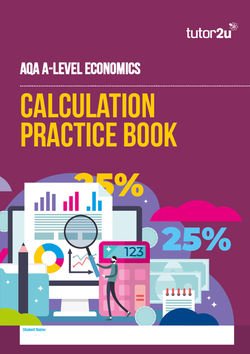Economics

Explore EconomicsSearch
Economics Topics

Linear Supply Function

The supply function can be written in the form of an equation

Qs = c + dP

• Where Qs is quantity supplied
• C = the level of supply independent of price
• P = the market price of the product
• d is the coefficient of price
• Supply for Product X = 10 + 2(P)
If the market price is £20, then Qs= 10 + 20 = 30 unitsEdexcel A Level Economics Synoptic Exercise Workbook (Paper 3)

• SKU: 02-4130-30240-03
• Printed Edition

• £7.95